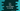# C++ program to find the sum of digits in a String## C++ program to find the sum of digits in a String:

In this program, I will show you how to find the sum of all digits in a string in C++. We will take the string from the user as input. Below are the steps we will use:

• Get the string from the user.
• Iterate through the characters of the string one by one.
• Check if the current character is digit or not. If it is a digit, add it to a sum variable. At the beginning of the program, we will assign this value 0.

### C++ program:

Below is the complete C++ program:

``````#include <iostream>

using namespace std;

int findSum(string str)
{
int sum = 0;

for (char ch : str)
{
if (isdigit(ch))
{
sum += ch - '0';
}
}
return sum;
}

int main()
{
string str;
cout << "Enter a string:" << endl;

getline(cin,str);
cout << "Sum :" << findSum(str) << endl;
}``````

### Explanation:

1. str is a string to hold the user input string.
2. Using getline, we are taking this string as input from the user.
3. findSum is used to find the sum of all numbers in a string. It uses one for loop to iterate through the characters of the string. If any character is digit, we are adding it to the variable sum. At the end of the loop, we are returning this value back.

### Sample Output:

``````hello world 123 !
Sum :6

Enter a string:
hello 1 world 23456 !
Sum :21``````